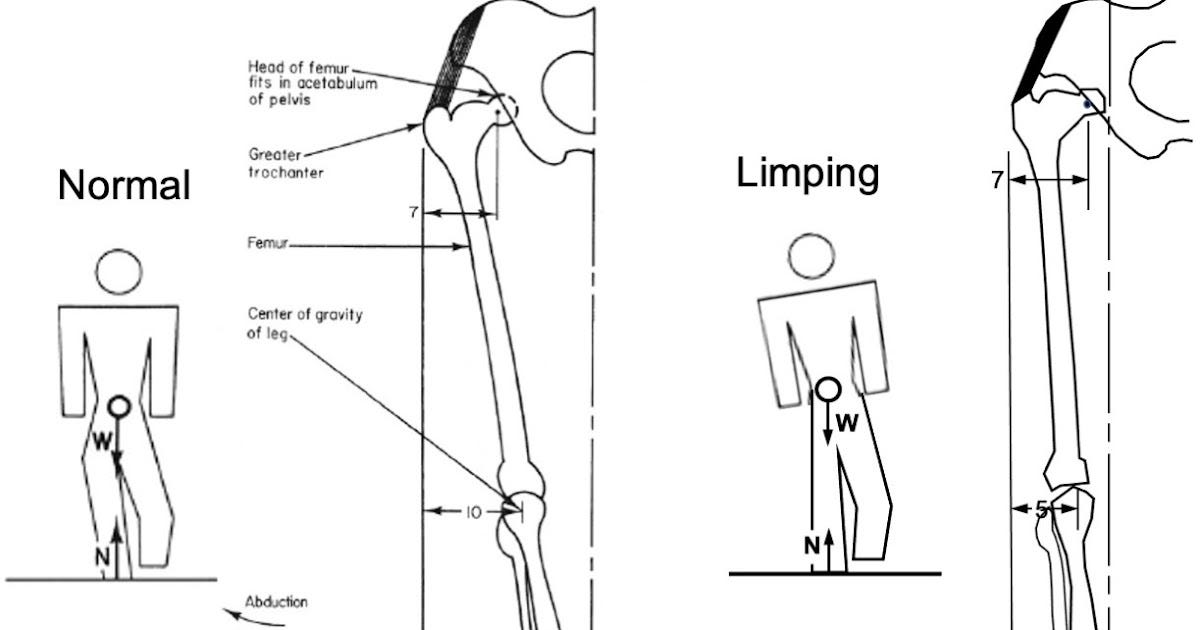# LimpingPertinent features of the anatomy of the leg: normal (left) and limping (right).

In Chapter 1 of Intermediate Physics for Medicine and Biology, Russ Hobbie and I discuss biomechanics. One of our most important examples is the force on the hip.

The forces in the hip joint can be several times a person’s weight, and the use of a cane can be very effective in reducing them.

Indeed, a cane is very useful, as Russ and I show in Section 1.8 of IPMB. But what if you don’t have a cane handy, or if you prefer not to use one? You limp. In this post, we examine the biomechanics of limping.

When you limp, you lean toward the injured side to reduce the forces on the hip. The reader can analyze limping in this new homework problem.

Section 1.8

Problem 11 ½. The left side of the illustration above analyzes normal walking and reproduces Figures 1.11 and 1.12. The right side shows what happens when you walk with a limp. By leaning toward the injured side you reduce the distance between the hip joint and the body’s center of gravity, and your leg is more vertical than in the normal case.

(a) Reproduce the analysis of Section 1.7 to calculate of the forces on the hip during normal walking using the illustration on the left. Begin by making a free-body diagram of the forces acting on the leg like in Figure 1.13. Then solve the three equations for equilibrium: one for the vertical forces, one for the horizontal forces, and one for the torques. Verify that the magnitude of the force on the hip joint is 2.4 times the weight of the body.

(b) Reanalyze the forces on the hip when limping. Use the geometry and data shown in the illustration on the right. Assume that any information missing from the diagram is the same as for the case of normal walking; For example, the abductor muscle makes an angle of 70° with the horizontal for both the normal and limping cases. Draw a free-body diagram and determine the magnitude of the force on the hip joint in terms of the weight of the body.

I won’t solve the entire problem for you, but I’ll tell you this: limping reduces the force on the hip from 2.4 times the body weight in the normal case to 1.2 times the body weight in the case of limping. No wonder we limp!

The main reason for the lower force when limping is the smaller moment arm. If we calculate torques about the head of the femur, then in the normal case the moment arm for the force that the ground exerts on the foot is 18–7 = 11 cm. When limping, this moment arm reduces to 9–7 = 2 cm. The moment arm for the abductor muscles (the gluteus minimus and gluteus medius) is the same in the two cases. Therefore, rotational equilibrium can be satisfied with a small muscle force when limping, although a large muscle force is required normally. The torque is a critical concept for understanding biomechanics.

What do you do if both hips are injured? When walking, you first lean to one side and then the other; you waddle. This reduces the forces on the hip, but results in a lot of swinging from side to side as you walk.

If you are having trouble solving this new homework problem, contact me and I’ll send you the solution.

Enjoy!

Originally published at http://hobbieroth.blogspot.com.

--

--